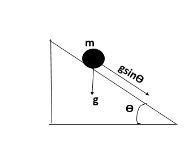# A solid cylinder and a hollow cylinder, both of them with equal mass and same external diameter are released from the same height at the same time on an inclined plane. Both roll down without any slipping. Which one will reach the bottom first?A. both together only when the angle of inclination of plane is $45{}^\circ$B. both togetherC. hollow cylinderD. solid cylinderVerified
208.5k+ views
Hint: The time taken to reach the bottom of an inclined plane by any of the body is given by the equation,

$t=\sqrt{\dfrac{2l\left( 1+\dfrac{{{K}^{2}}}{{{R}^{2}}} \right)}{g\sin \theta }}$

Here t is the time taken to reach the bottom of inclined plane, I is the length of inclined plane, R is the radius of any of the cylinder, g the acceleration due to gravity is and $\theta$ the angle of inclination.
This equation should be used for both the cylinders in order to get the answer.

An object kept on a tilted surface will sometimes slide down the surface.As we know, the time taken to reach at the bottom of inclined plane is given by the equation,

$t=\sqrt{\dfrac{2l\left( 1+\dfrac{{{K}^{2}}}{{{R}^{2}}} \right)}{g\sin \theta }}$
In this equation, the term $\dfrac{{{K}^{2}}}{{{R}^{2}}}$is decided by the material used.
For a solid cylinder,
$\dfrac{{{K}^{2}}}{{{R}^{2}}}=\dfrac{1}{2}$
Therefore,
${{K}^{2}}=\dfrac{{{R}^{2}}}{2}$
For a hollow cylinder,
${{K}^{2}}={{R}^{2}}$
Substituting both these in the time taken equation will tell that the solid cylinder is taking a shorter time. Therefore, the solid cylinder will reach the bottom first.

So, the correct answer is “Option C”.

Note:
Objects have the tendency to accelerate down inclined planes because of an unbalanced force. This is restricted for an extent by the frictional force over there on that object. In order to understand this kind of motion, it is mandatory to analyse the forces acting upon an object on an inclined plane.